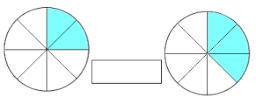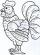# Quotient and division

Find the quotient of 3/4 and 1/4.

k =  3

### Step-by-step explanation:Did you find an error or inaccuracy? Feel free to write us. Thank you!Tips to related online calculators
Need help to calculate sum, simplify or multiply fractions? Try our fraction calculator.

## Related math problems and questions:

• Quotient and divisionWhat is the quotient of 2/9 and 1/8?
• A quotientWhat is the quotient of 3/10 divided by 2/4 as a fraction?
• Quotient 3If the quotient of 8/13 and 2 is subtracted from the product of 1 3/4 and 8/21, what is the difference?
• The quotientThe quotient of g and 55 is the same as 279. What is g?
• Find the 11Find the quotient of 229.12 and 12.32
• QuotientDetermine the quotient (q) and the remainder (r) from division numbers 100 and 8. Take the test of correctness.
• What is 11What is the quotient of Three-fifths and 1 Over 10?
• Quotient and productIf the quotient of [8/5 divided by 8/10] is added to the product of [8/14 x 7/12 x 3/8], what is the sum?
• QuotientFind quotient before the bracket - the largest divisor 51 a + 34 b + 68 121y-99z-33
• Evaluate 11Multiply the quotient of 6 and 2 by 3, then add 1. Add 1 and 6, then divide by 2 and multiply by 3. Divide 6 by the product of 2 and 3, then add 1.
• Product of two fractionsProduct of two fractions is 9 3/5 . If one of the fraction is 9 3/7. Find the other fraction.
• Result and remainderAfter dividing the unknown number by the number 23, the quotient 11 and the remainder 4 are formed. Find an unknown number.
• SixteenSixteen minus the quotient of 12 and what number is thirteen?
• Evaluate expr with 2 varsIf a=6 and x=2, find the value of (2ax + 7x- 10) /(4ax-3a-2)
• Numbers divisionWith what number should be divided mixed number 2 3/4 to get 11/12?
• The length 6The length of 12 pipes is 10 1/2 meters. (1) find the length of one pipe (2) also find the length of 7 pipes
• Reminder and quotientThere are given the number C = 281, D = 201. Find the highest natural number S so that the C:S, D:S are with the remainder of 1,